Eth: 0x00cce8E2e56a543abc084920eee3f88eFD0921ea

## Monday, February 13, 2012

### Modeling Gravity with Matlab

It seemed a bit cumbersome to organize all this code and information on a blog so I've move to the Spaceience Website hosted by google. I also put some more explanations in there on the simpler to implement models (non-ODE45).

Today there are some amazingly neat computer programs that can allow students at the high school and college level to easily extend what they have learned in the classroom to real applications. However, it's not as if you're teachers will walk you through this, they have better things to do! Today I'll walk you through creating a very simple gravity simulator in Matlab using the basic equations of gravitational attraction that you know and adding in a little Matlab coding to make it dance. We will only be using the sun and the earth in this first model but it can easily be extended to include whatever planets you like.

What You Need
The only thing you'll need to do this project is
• a student copy of Matlab 2007 or better
• access to Wikipedia to look up terms you might have forgotten

Review the System Information
Matlab is a very smart program. If you tell it the position, velocity and acceleration of a particle, it can predict where that particle will end up. A similar example is if we were in a car at a stop light and as soon as the light turned green we would start constantly accelerating at 5 m/s^2, you could calculate our position in ten seconds. In this case, our initial position at the stoplight is our x position =0. Our initial velocity is also = 0 since we start from a stop. Our constant acceleration, however, is = 5 m/s^2. For the Earth/Sun system, we can easily find the position and velocity of the Earth at any point in it's orbit. The acceleration is the hard part since it is constantly changing. If we can find a general expression for the acceleration, we can give the position, velocity and acceleration to Matlab and Matlab will do that calculation you did to find the cars position a thousand times over in the blink of an eye. It has to do the calculation lots of times because the acceleration is changing based on the position of Earth.
First thing we want to do before coding up a model in matlab is to understand the basic equations at work. Starting from the first principles of Newton's Laws governing gravitational attraction we want to understand the acceleration of the Earth as it goes around the sun.From Wikipedia: Gravitational Constant
Starting from this principle, we will focus on finding the acceleration of the Earth.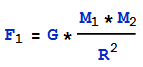This is telling us that the force gravity exerts is equal to the universal gravitational constant times the mass of of the first object, times the mass of the second object, divided by the radius between them squared. For our purposes we will set G=1.4879*10^(-34) in units of AU^3/(kg*Day^2). The reasons for these units is because we want it to work easily with the initial position and velocity data we will acquire from the JPL ephemeris database.

Force can also be expressed as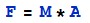. Combining these two equations we find the following: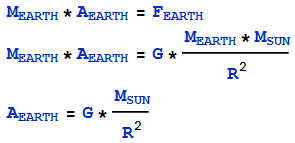Similarly for the Sun we can say: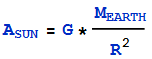So we have found an expression for the acceleration of the sun and the acceleration of Earth. For this simple model we will assume that the amount of movement that the sun experiences from earth is so small it basically stays still. This means we will neglect the acceleration of the sun from our calculations which will make life much simpler. As always, you can add this in later.
Note: Mass sun is a constant, G is a constant, R is NOT a constant

Position and Velocity Data from JPL
JPL keeps a database of the predicted position and velocity of every cataloged object in our solar system. This includes asteroids! We need to select a date, and search the database to tell us our initial conditions which will later be put into Matlab. Go to the JPL HORIZONS web-interface. We only need to find the inial position of the earth for the date specified. This is because we are assuming the sun is at [0,0,0] and has no acceleration or velocity. Make sure the ephemeris type is Vectors. The target body should be Earth (Geocenter) as opposed to the Earth-Moon barycenter which is the center of the Earth-Moon system. We will use a start date of February 12, 2012. A step size of 10 days will give us the position on the 12th and the 22nd.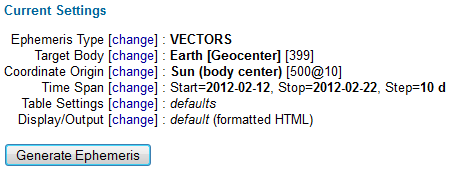JPL HORIZONS Web-Interface

Then we click generate and we get the following data: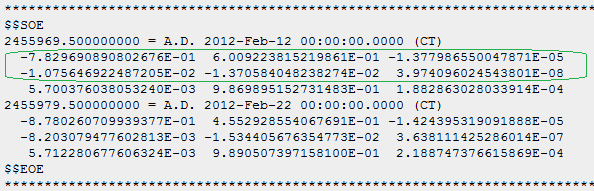The data we will use is highlighted in green. Make sure to read the table at the top of the Ephemeris to make sure you understand what the units are. For our example, in the green box, the top row is the [X,Y,Z] position of Earth in AU. The second row below that is the [Vx,Vy,Vz] velocities of Earth in AU/Day.

Build it in Matlab
Lets review the information we've collected:
• Sun - position, velocity, acceleration all assumed to be zero
• Earth - position and velocity from JPL, have a function for acceleration in terms of radius
Now that we have all our starting information we can build the Matlab simulator. Open up Matlab and create a new script file saved as "SimpleGravity.m". In this we will build our program. We will need to also build a separate function in another script file. Create a new script file and save it as "Propagator.m". SimpleGravity will call Propagator when we run it.

Components of SimpleGravity.m
•  Input the time over which we'd like to see the simulation run as well as the size of the time step we would like to use. A smaller time step means more processing is required.
• Create some global variables
• Input initial conditions for the Sun from our assumptions [0,0,0] position, [0,0,0] velocity
• Input initial conditions for the Earth from JPL
• Call the process ODE45 on the function Propagator.m function
• Plot the results

Components of Propagator.m

This part is where you'll need to look up some Matlab functions and familiarize yourself with Matlab programming. I could walk you through every function call but that wouldn't be exciting. I have included a pdf document detailing the final result of each of the above steps. However, I suggest that you try to write it up yourself first. It's ok if you don't know how the ODE45 command works, just try and get the basic commands down before using the cheat sheet. ODE45 takes your current position, velocity and acceleration and calculates what your next position and velocity will be at some timestep later specified by you. (It uses a Runge-Kutta method for you math geeks). Matlab is wonderfully useful for all sorts of calculations. If you're an engineering student, I really suggest you take the time to really learn and understand this program.

Matlab Code
SimpleGravity.m
Propagator.m

Make sure that you put them in the same directory and execute SimpleGravity.m. Change the result by making the step size smaller or increasing the number of days the final time.

If you want to check how well your simulator works, set finaltime to 10 days. Look at the last line of finalposition, the first three numbers represent the last x,y,z position of Earth. Compare this against the position given by JPL from our initial data show in the picture above.

Feedback
Send Feedback Please! Hope you have been inspired.

Allan said...

Thanks for the post, very interesting and very much appreciated.

I'm hoping to use matlab to eventually create a model of the solar system and I could use a lot of matlab help (I've just started using it). Would you be able to help with a few questions? If so do you have a contact email address?

Thanks again,
Allan.

Eliot said...

Allan: Sure you can contact me at nonstored@gmail.com

Allan said...

Hello again,

I attempted to send you an email but it's bouncing back from the server.

Do you have an alternative address or could you email me and I'll reply? (alland2000@hotmail.com)

Thanks again,
Allan.

Shahin said...
This comment has been removed by the author.
Unknown said...

Thanks for the explanation, it helped me a lot. I think it has an error when ploting though, you're ploting the velocities not the positions.